# Expectation Maximization algorithm for feather data clustering

#### Introduction

The primary focus as a Seismic Survey Specialist is the identification of tidal current patterns in seismic surveys and to exploit them in order to optimize sail line selection in 4D surveys. The feather data – i.e. the bearing of the seismic cables to the course made good of the vessel – collected can be analysed for hidden patterns. These patterns can help with the forecasting of tidal currents for  subsequent surveys.

The following is the preliminary draft of a report I performed on the usage of statistical packages to conduct pattern analysis. Feel free to comment on any aspects of this work.

#### Identification of clusters in univariate data

The current report exhibits the clustering of Gaussian mixtures in univariate data using R to perform the processing. It was identified and postulated (initially by Ewan Nicolson) that certain distributed data might be produced by mixtures of Gaussian Normals when collected over a time-period. Certain normal distributions began to emerge when looking at feather data [see Figure 1].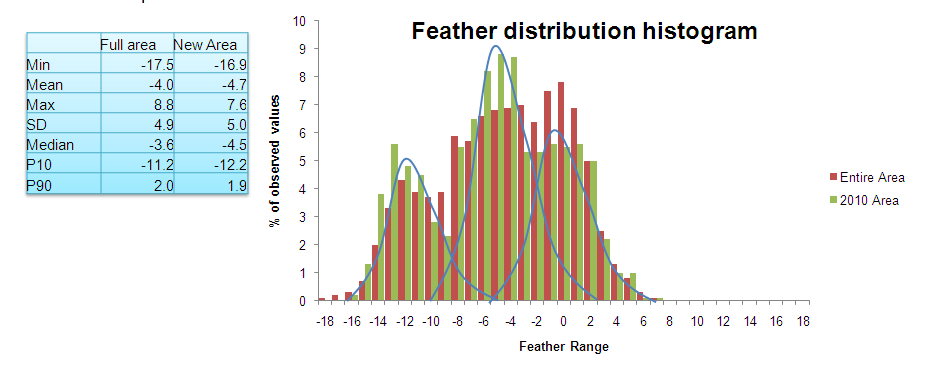Figure 1: Apparent gaussian distributions in the feather dataset

We wanted to show whether this apparent emergence was within the levels of acceptable confidence. To do this classification, the best procedure to provide accurate determination of individual mixtures was the Expectation Maximization (EM) Algorithm. The EM is a method for finding the maximum likelihood or maximum a posteriori Bayesian estimates of parameters in statistical modeling. The advantage of this technique is that the EM algorithm performs an unsupervised clustering method, i.e. it doesn’t require a training phase, based on mixture models. It follows an iterative approach, sub-optimal, which tries to find the parameters of the probability distribution that has the maximum likelihood of its attributes.

#### Case study

The case study which gave rise to this hypothesis was a seasonal feather distribution and the similarities it exhibited between consecutive years. In Figure 1 the feather distribution of two datasets is overlaid. In red the values from an area sampled in 2008 and in green a subset of the initial area sampled in 2010. The distinct tri-modal fit is overlaid in blue to denote the different trends identified with this sample.

To determine how many clusters emerged and where they were situated, the data was imported into R as a 1-dimensional vector. The initialization of the EM algorithm first determined the number of components in the Gaussian mixture model in order to use as starting points for the subsequent convergence. This stage calculated the following Bayesian Information Criterion for the parameterized models given the loglikelihood, the dimension of the data, and the number of mixture components in the model [see Figure 2].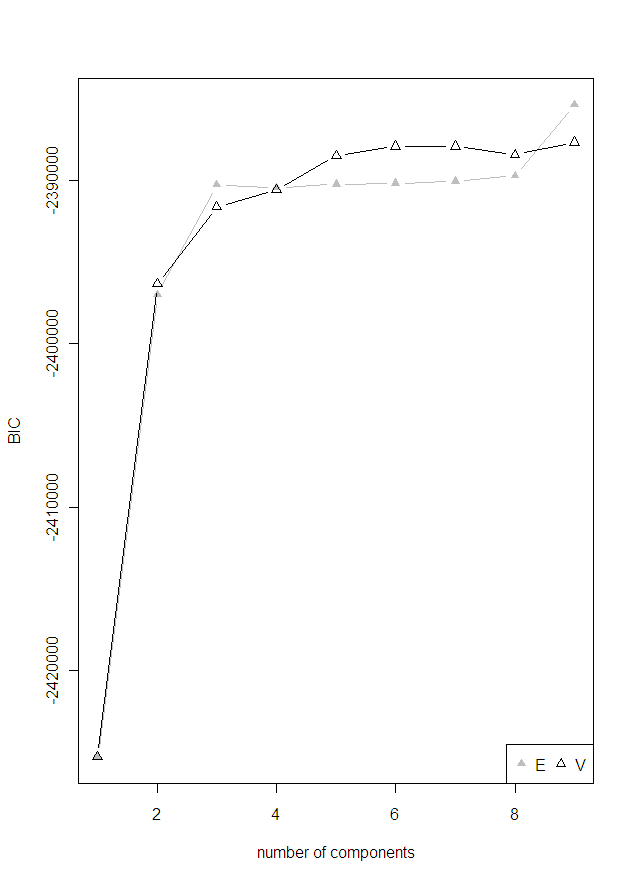Figure 2: Bayesian Information Criterion

The first time the two univariate mixture models (E(qual variance), V(ariable variance)) converge is when there are 4 clusters. The algorithm though misses that point and instead selects automatically the default maximum which is 9 clusters.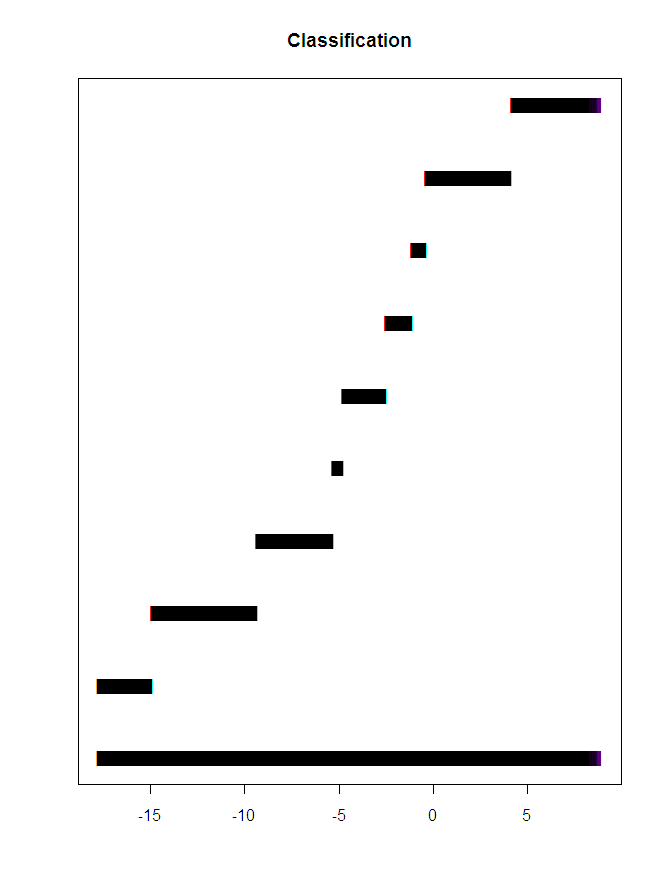Figure 3: Classification for 9 clusters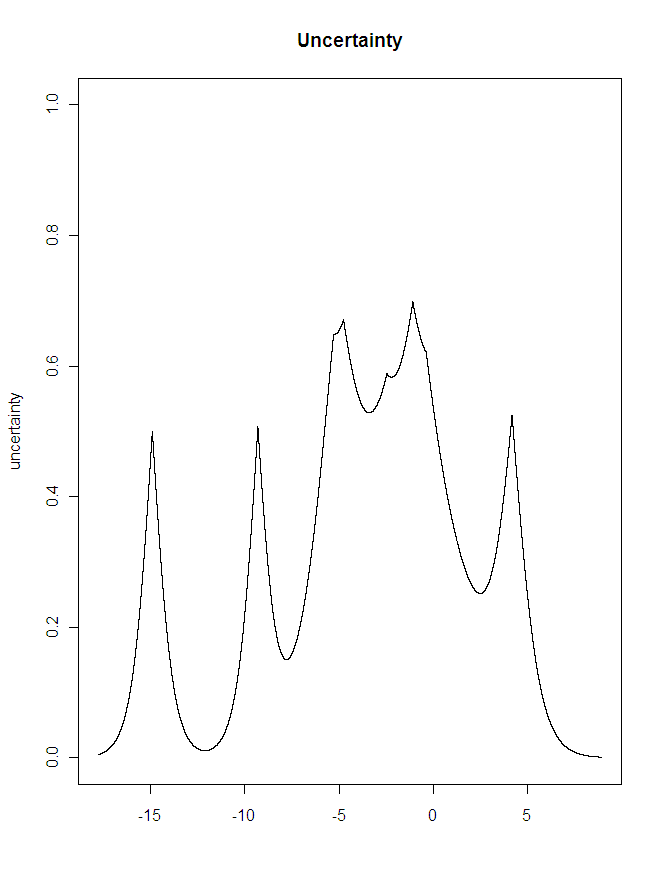Figure 4: Uncertainty for 9 clusters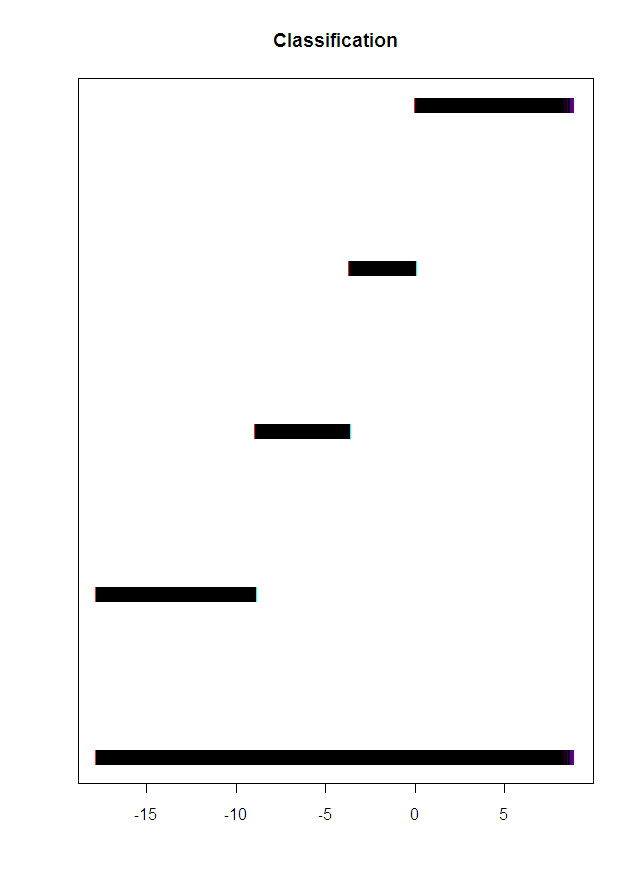Figure 5: classification for 4 clusters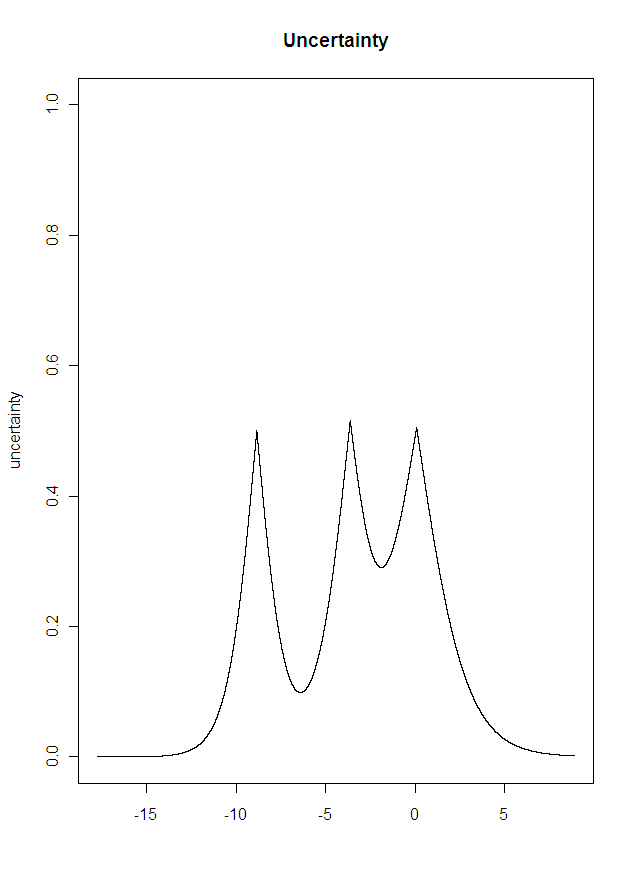Figure 6: Uncertainty for 4 clusters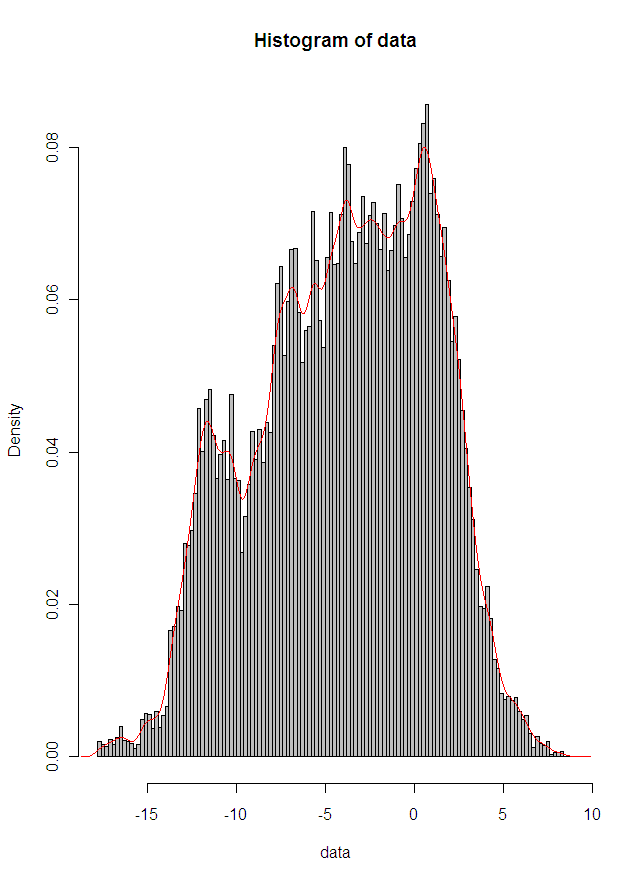Figure 7: Histogram overlaid with density function

The process then is running a convergence algorithm in order to identify the parameters of each mixture as defined by BIC [see Figure 3]. This model seems to be identifying the mixtures we were expecting but also a verbose amount of additional smaller ones which are irrelevant at this stage. When the EM algorithm is run with a manual specification of 4 clusters, then the underlying mixtures emerge much better. The algorithm in this case has clearly identified the four evident mixtures in the feather dataset [see Figure 5] as they are shown in the density function of the distribution in Figure 7.

#### Future Work

The procedure seems to be working correctly in identifying the underlying clusters in feather data. The package used -mclust - needs more exploration in order to produce the mixture parameters (mean, variance) for each individual mixture identified and also to produce some overlaid plots of the clusters on top of the distribution.

 http://en.wikipedia.org/wiki/MCLUST